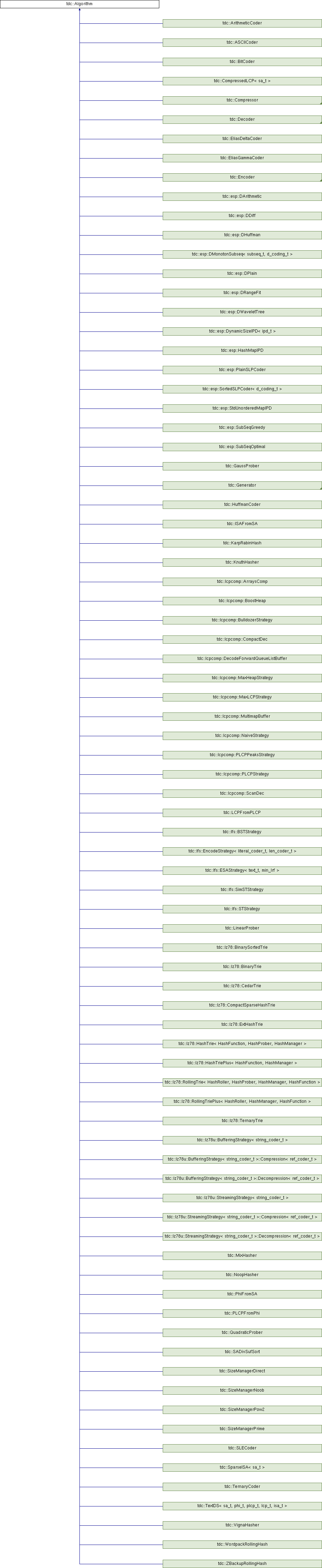tudocomp – The TU Dortmund Compression Framework
tdc::Algorithm Class Reference

Interface for algorithms. More...

`#include <Algorithm.hpp>`

Inheritance diagram for tdc::Algorithm:## Public Member Functions

virtual ~Algorithm ()=default

Algorithm (Algorithm const &)=default

Algorithm (Algorithm &&)=default

Algorithmoperator= (Algorithm const &)=default

Algorithmoperator= (Algorithm &&)=default

Algorithm (Env &&env)
Instantiates an algorithm in the specified environment. More...

Envenv ()

const Envenv () const

## Detailed Description

Interface for algorithms.

This is the base for classes that use an environment (Env) to receive options or communicate with the framework in different ways.

Algorithms are required to implement also a static function `meta()` that returns a Meta object, containing information about the algorithm.

Definition at line 15 of file Algorithm.hpp.

## ◆ ~Algorithm()

 virtual tdc::Algorithm::~Algorithm ( )
virtualdefault

## ◆ Algorithm() [1/3]

 tdc::Algorithm::Algorithm ( Algorithm const & )
default

## ◆ Algorithm() [2/3]

 tdc::Algorithm::Algorithm ( Algorithm && )
default

## ◆ Algorithm() [3/3]

 tdc::Algorithm::Algorithm ( Env && env )
inline

Instantiates an algorithm in the specified environment.

Parameters
 env The environment for the algorithm to work in.

Definition at line 31 of file Algorithm.hpp.

## ◆ env() [1/2]

 Env& tdc::Algorithm::env ( )
inline

Returns
The environment that the algorithm works in.

Definition at line 51 of file Algorithm.hpp.

## ◆ env() [2/2]

 const Env& tdc::Algorithm::env ( ) const
inline

Definition at line 52 of file Algorithm.hpp.

## ◆ operator=() [1/2]

 Algorithm& tdc::Algorithm::operator= ( Algorithm const & )
default

## ◆ operator=() [2/2]

 Algorithm& tdc::Algorithm::operator= ( Algorithm && )
default

The documentation for this class was generated from the following file: